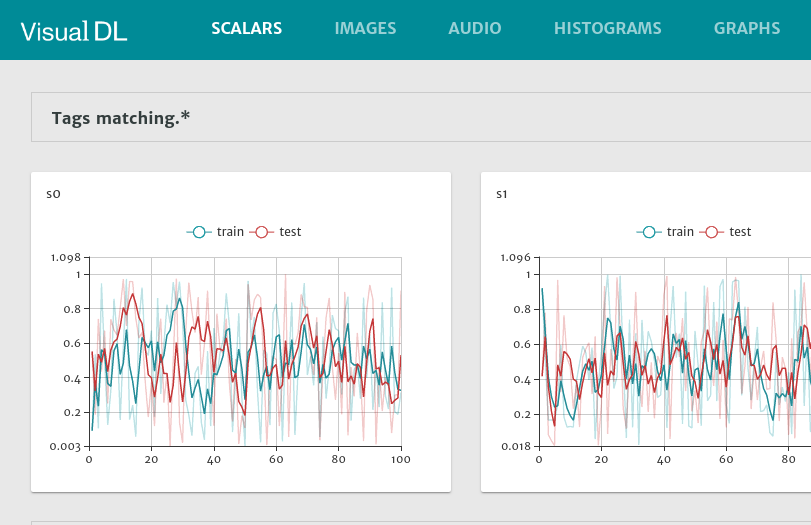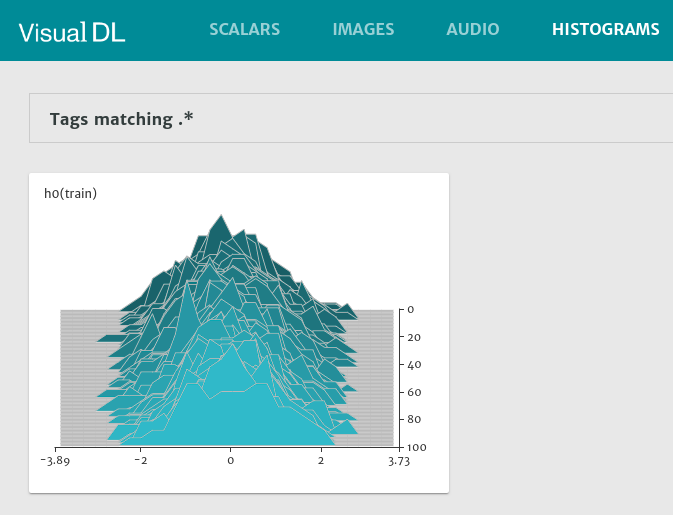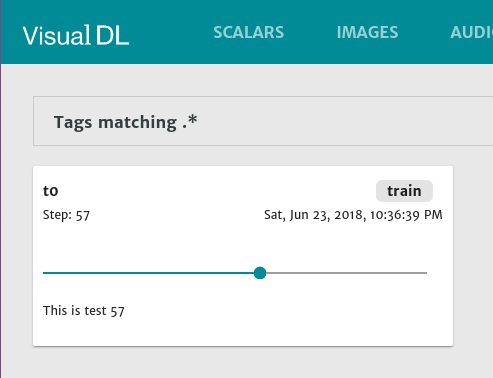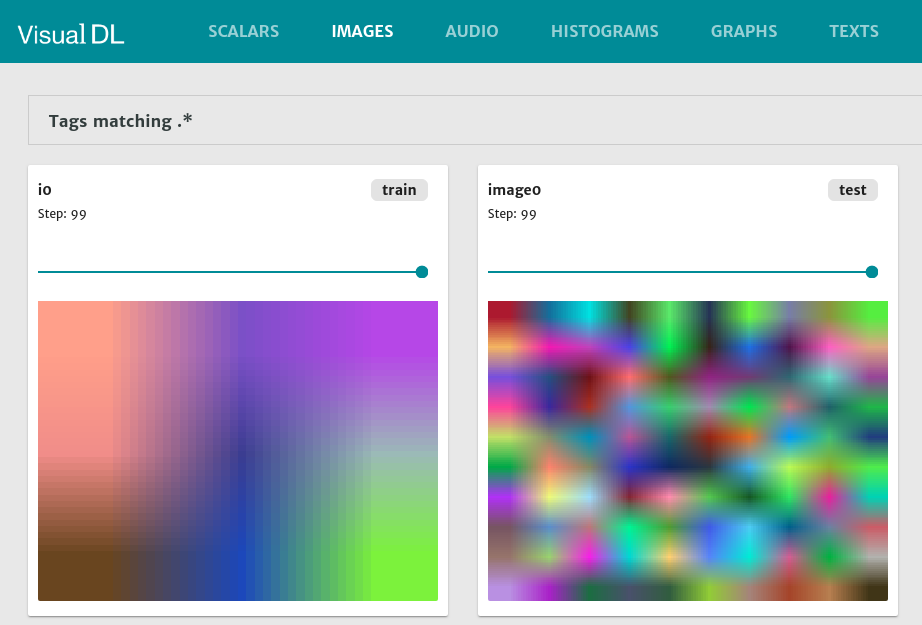9

3

1

1

# VisualDL.jl

This package provides a julia wrapper for VisualDL, which is a deep learning visualization tool that can help design deep learning jobs.

Currently, the wrapper is written on top of the Python SDK of VisualDL by PyCall. I have tried to write the wrapper on top of the C++ SDK by leveraging CxxWrap.jl. But unluckily a strange error encountered. Hopefully I'll figured it out later and swap the backend into C++.

## Install

• First, install the Python client of VisualDL. Checkout here for a detailed guide.

• Then add this package as a dependent(only tested on Julia v0.7).

`(v0.7) pkg> add VisualDL`

## Usage

First, initial the logger.

``````using VisualDL

train_logger = VisualDLLogger("tmp", 1, "train")
test_logger = as_mode(train_logger, "test")
``````

### Scalar

``````for i in 1:100
with_logger(train_logger) do
@log_scalar s0=(i,rand()) s1=(i, rand())
end

with_logger(test_logger) do
@log_scalar s0=(i,rand()) s1=(i, rand())
end
end
``````### Histogram

``````for i in 1:100
with_logger(train_logger) do
@log_histogram h0=(i, randn(100))
end
end
``````### Text

``````for i in 1:100
with_logger(train_logger) do
@log_text t0=(i, "This is test " * string(i))
end
end
``````### Image

``````for i in 1:100
with_logger(train_logger) do
@log_image i0=([3,3,3], rand(27) * 255)
end
end

for i in 1:100
with_logger(test_logger) do
@log_image image0=rand(10, 10, 3) * 255
end
end

# force save and sync
save(train_logger)
save(test_logger)
``````Finally, run `visualDL --logdir ./tmp` in current dir. Then launch the visualdl service and watch the above pictures in browser. The default url is `http://localhost:8040`:

## TODO

• [x] More documentation
• [x] ~~Add `LogReader`~~ and tests
• [x] Precompile
• [x] Travis
• [x] Make Release
• [ ] Move out the `start_sampling` and `finish_sampling` from `@log_image` and `@log_audio`

06/23/2018